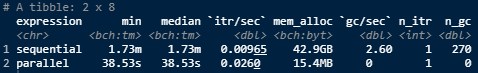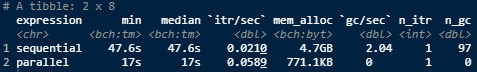# Disposition Effect in Parallel

To load the package simply use the usual library function.
This tutorial requires also some well-known packages that allows for parallel computations in R.

library(dispositionEffect)
library(dplyr)
library(foreach)
library(parallel)
library(doParallel)
library(future)
library(furrr)
library(bench)

# Data

The disposition effect analysis is performed on two fundamental types of data frames:

• portfolio transactions, that is all the financial transactions an investor did during a specific period of time. A single transaction is made up of 6 features: the investor id, the asset id, the type of the transaction (it can be a buy or a sell), the traded quantity, the traded price, and the datetime.

• market prices, that is the prices found on the stock markets for each traded asset and each transaction datetimes.

# The Problem

Usually, investor’s transactions datasets may be huge in size. Moreover, the dataset of market prices may grow exponentially since it has to contain the market prices for each traded asset on each trading datetime.

This may cause the computation of gains and losses through the main interface of the package (portfolio_compute) to take few minutes in order to produce the output data frame.

This computational inefficiency is particularly due to the size of the market price dataset, since for every transaction we need to look for the closest available market price at that datetime for each asset.

For this reason, we used few parallel methodologies to improve the computation time on large datasets.

# The Solution: Parallel Computing

## 1) Parallel porfolio_compute

Usually, you may want to apply the computations on many different investors. To do that one can simply build a function that loops on a list containing the transactions portfolios. The first solution to this problem is to develop a parallel version of portfolio_compute that allows to compute results on many investors in parallel, taking advantage of two very useful R packages: future and furrr.

A very simple version of the function would look like this.

portfolio_compute_parallel <- function(portfolio_transactions, market_prices, plan = NULL, ...) {

investors_id <- purrr::map_chr(portfolio_transactions, ~purrr::pluck(., "investor"))
portfolio_compute_safe <- purrr::safely(portfolio_compute)

if (is.null(plan)) {
ncores <- future::availableCores()
# if there are more than 2 cores than use parallel computing
# otherwise use sequential computing
# RULE: be polite, always leave at least 1 free core
if ((ncores - 1) > 1) {
new_plan <- "multisession" # since I am testing on Windows
} else {
new_plan <- "sequential"
}
} else {
new_plan <- plan
}

old_plan <- future::plan(strategy = new_plan)

res <- furrr::future_map(
portfolio_transactions,
portfolio_compute_safe,
market_prices,
...)

res <- purrr::transpose(res)$result names(res) <- investors_id future::plan(old_plan) # set back the old plan return(res) } This way you simply need to store all the different investor’s portfolios of transactions in a list and pass it to portfolio_compute_parallel as first argument. Now let’s try it on the DEanalysis dataset to see if there is any computational improvement. trx <- DEanalysis$transactions %>%
dplyr::group_by(investor) %>%
dplyr::group_split()
mkt <- DEanalysis$marketprices res <- bench::mark( "sequential" = portfolio_compute_parallel(trx, mkt, plan = "sequential"), "parallel" = portfolio_compute_parallel(trx, mkt, plan = "multisession") ) res$expression <- c("sequential", "parallel")
res[, 1:8]As you can see, the benefits of parallel code are clear even on this small sample dataset.

## 2) Separate files

Although the first solution may be enough with many small investors, when things get bigger it may be better to physically separate transactions and market prices for each investor.

This way, a single .RData that contains both the transactions and the market prices for a single investor is created. Once this process is finished, one can simply perform the computations with the usual portfolio_compute loading the needed file sequentially or in parallel by means of the foreachpackage.

First let’s store every investor’s datasets into its own file. To do this we can make use of the dispositionEffect:::generate_data internal function. In this way, we are able also to optimize the market price dataset, since for each investor we can save only the market prices of his traded assets for that specific period of trading.

cl <- parallel::makeCluster(parallel::detectCores())
doParallel::registerDoParallel(cl)
foreach::foreach(i = seq_along(trx), .errorhandling = "pass") %dopar% {

transactions <- dplyr::arrange(trx[[i]], datetime)
mrkt_prices <- dplyr::filter(
mkt,
asset %in% unique(transactions$asset) & datetime <= max(transactions$datetime)
)
df <- dispositionEffect:::generate_data(transactions, mrkt_prices, subset = TRUE)
nm <- paste0("INV", i, ".RData")
save(df, file = nm)

}
parallel::stopCluster(cl)

Now, we can build the function to perform computations loading each single file sequentially or in parallel.

portfolio_compute_onfiles <- function(files, plan = "sequential") {

if (plan == "sequential") {

res_list <- vector(mode = "list", length = length(files))
for (i in seq_along(files)) {
tmp_res <- tryCatch(
dispositionEffect::portfolio_compute(
portfolio_transactions = df$transactions, market_prices = df$marketprices
),
error = function(e) "Error"
)
res_list[[i]] <- tmp_res # save results
rm(df, tmp_res)
}

} else {

cl <- parallel::makeCluster(parallel::detectCores())
doParallel::registerDoParallel(cl)
res_list <-
foreach(i = seq_along(files), .errorhandling = "pass") %dopar% {
tmp_res <- tryCatch(
dispositionEffect::portfolio_compute(
portfolio_transactions = df$transactions, market_prices = df$marketprices
),
error = function(e) "Error"
)
}
parallel::stopCluster(cl)

}

return(res_list)

}
files <- list.files(pattern = ".RData") # list all single .RData

res <- bench::mark(
portfolio_compute_onfiles(files, plan = "sequential"),
portfolio_compute_onfiles(files, plan = "multisession")
)

res\$expression <- c("sequential", "parallel")
res[, 1:8]# Conclusions

As expected, parallel computation coupled with load in memory small portion of datasets is the optimal solution.

Moreover, sometimes a little gain in computation time can be obtained by setting the exact_market_prices argument of portfolio_compute equal to TRUE. This way, the closest market price search is performed on the very same transaction datetime, but it requires that the market price dataset contains all the prices for each asset at each single transaction datetime.

This tests have been performed on a 4 core, 8GB RAM, Windows machine

#>                _
#> platform       x86_64-pc-linux-gnu
#> arch           x86_64
#> os             linux-gnu
#> system         x86_64, linux-gnu
#> status
#> major          4
#> minor          2.0
#> year           2022
#> month          04
#> day            22
#> svn rev        82229
#> language       R
#> version.string R version 4.2.0 (2022-04-22)
#> nickname       Vigorous Calisthenics

For more tutorials on disposition effect visit dispositionEffect.